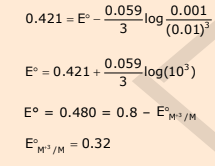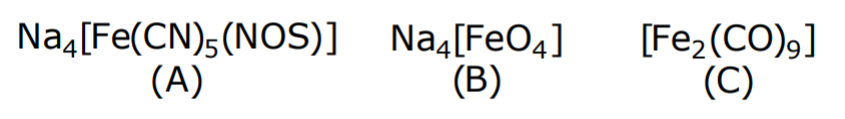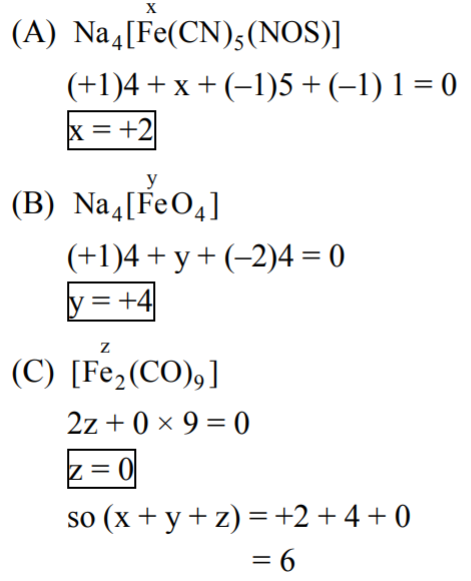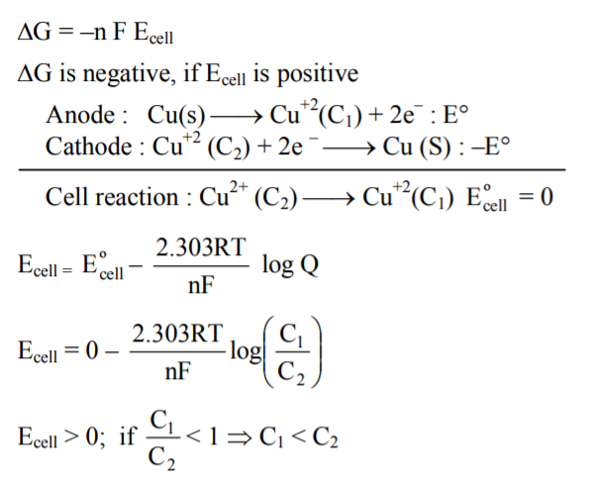Filter By

## All Questions

#### To find the standard potential of M3+/M electrode, the following cell is constituted: Pt/M/M3+(0.001 mol L−1)/Ag+(0.01 mol L−1)/Ag \ The emf of the cell is found to be 0.421 volt at 298 K.  The standard potential of half reaction M3++3e− → M at 298 K will be : Given  EAg+/Ag at 298 K=0.80 Volte Option: 1  0.38 Volt Option: 2  0.32 Volt Option: 3 1.28 Volt Option: 4 0.66 Volt#### The oxidation number of potassium in respectively is : Option: 1Option: 2Option: 3Option: 4(In peroxide, the oxidation state of oxygen is -1)

(In superoxide, the oxidation state of oxygen is -1/2)

Therefore, Option(1) is correct.

## Crack CUET with india's "Best Teachers"

• HD Video Lectures
• Unlimited Mock Tests
• Faculty Support#### Given that the standard potentials (Eo) of Cu2+|Cu and Cu+|Cu are 0.34V and 0.522V respectively, the Eo of Cu2+|Cu+ is: Option: 1Option: 2Option: 3Option: 4Given,

We need to find out the electrode potential of the reaction

Reaction (3) can be obtained by carrying out the operation (1) - (2)

Thus, we can write

Therefore, Option(2) is correct.

#### For an electrochemical cell  the ratio  When this cell attains equilibrium is _____.   Option: 1Option: 2 1.11 Option: 3 7.15 Option: 4 3.14

As we have learnt,

Nernst equation is given as

Now, the chemical reaction occuring in the cell is given as

Hence, the option number (1) is correct.

## Crack NEET with "AI Coach"

• HD Video Lectures
• Unlimited Mock Tests
• Faculty Support#### The equation that is incorrect is: Option: 1Option: 2Option: 3Option: 4Both sides are not equal.

Therefore, Option(1) is correct.

## Crack JEE Main with "AI Coach"

• HD Video Lectures
• Unlimited Mock Tests
• Faculty Support#### Potassium chlorate is prepared by the electrolysis of in basic solution  If only 60% of current utilized in the reaction, the time (hr) required to produce 10 g of using a current of 2A is ___

The reaction occurs as follows:

Current efficiency = 60%

Now, moles of

Thus, moles of  (assuming 100% efficiency)

Thus, actual moles of

Thus, t = 39387.76 sec. = 10.94 hours.

Thus, time required is approximately 11 hours.

#### The redox reaction among the following is: Option: 1 Formation of ozone from atmospheric oxygen in presence of sunlight Option: 2 Redox ofwithOption: 3 Combination of dinitrogen with dioxygen of 2000K. Option: 4 Reaction ofwithshowing redox reaction, both oxidation and reduction.

Therefore, Option(3) is correct.

## Crack CUET with india's "Best Teachers"

• HD Video Lectures
• Unlimited Mock Tests
• Faculty Support#### The oxidation states of iron atoms in compounds (A), (B) and (C), respectively,  are x, y and z. The sum of x, y and z is______.#### For the given cell; change in Gibbs energy  is negative, if :  Option: 1Option: 2Option: 3Option: 4We know this formulaOnlyfollowing the above condition.

Therefore, the correct option is (4).